# 选股公式实战解析

2019-06-25 21:17:54 技术分析知识库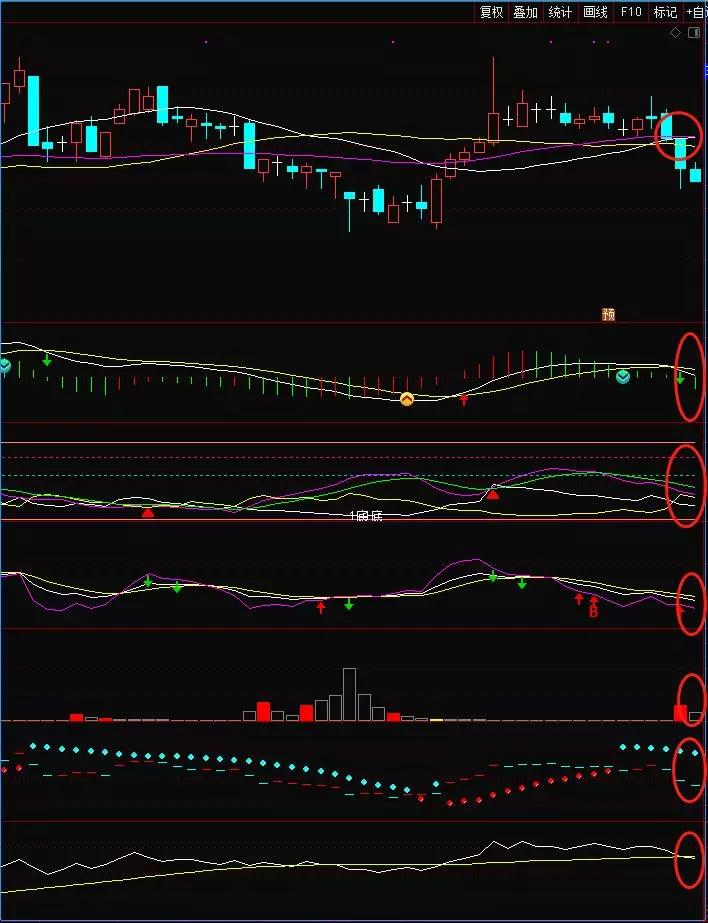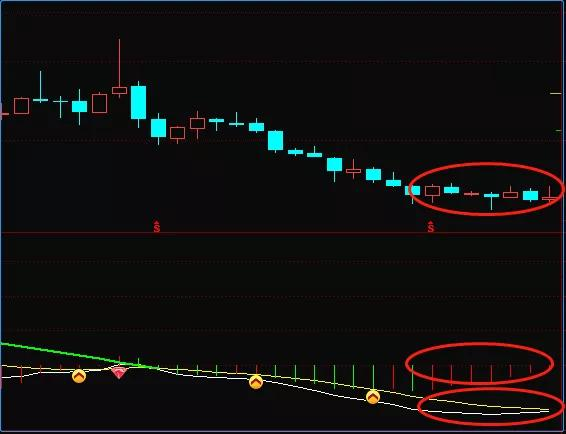A0:=0.01*AMOUNT/V;
DIF:=(EMA(A0,12)-EMA(A0,26));
DEA:=(EMA(DIF,9));
MACD:=(DIF-DEA)*2;
MM:=100*(C-REF(C,2))/REF(C,2);
T:=EVERY(DEA<REF(DEA,1) AND DEA<0 AND DEA<MACD AND ABS(MM)<10,3);
BARSLAST(LONGCROSS(DIF,REF(DIF,1),5))<4 AND T;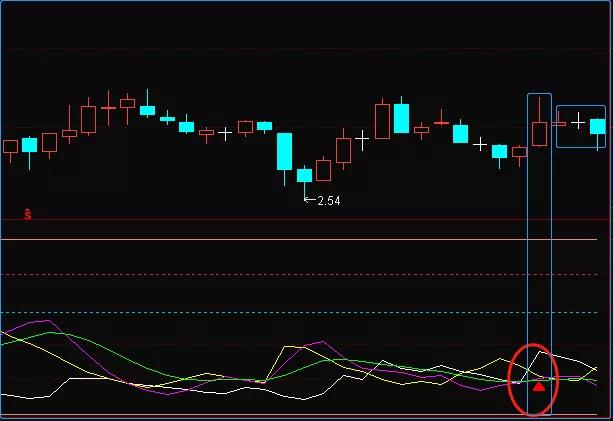MTR:=EXPMEMA(MAX(MAX(HIGH-LOW,ABS(HIGH-REF(CLOSE,1))),ABS(REF(CLOSE,1)-LOW)),14);
HD:=HIGH-REF(HIGH,1);
LD:=REF(LOW,1)-LOW;
DMP:=EXPMEMA(IF(HD>0&&HD>LD,HD,0),14);
DMM:=EXPMEMA(IF(LD>0&&LD>HD,LD,0),14);
PDI:=DMP*100/MTR;
MDI:=DMM*100/MTR;
N1:=BARSLAST(CROSS(PDI,MDI));
EXIST(T,3);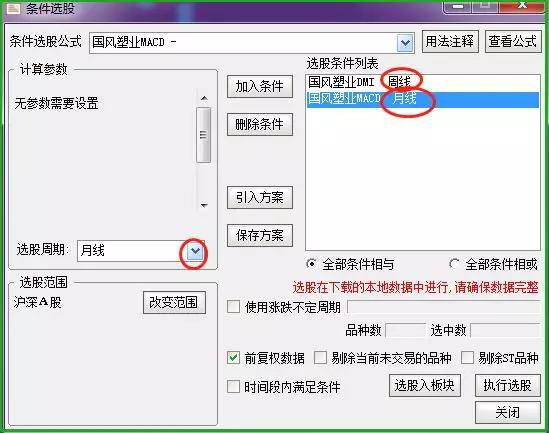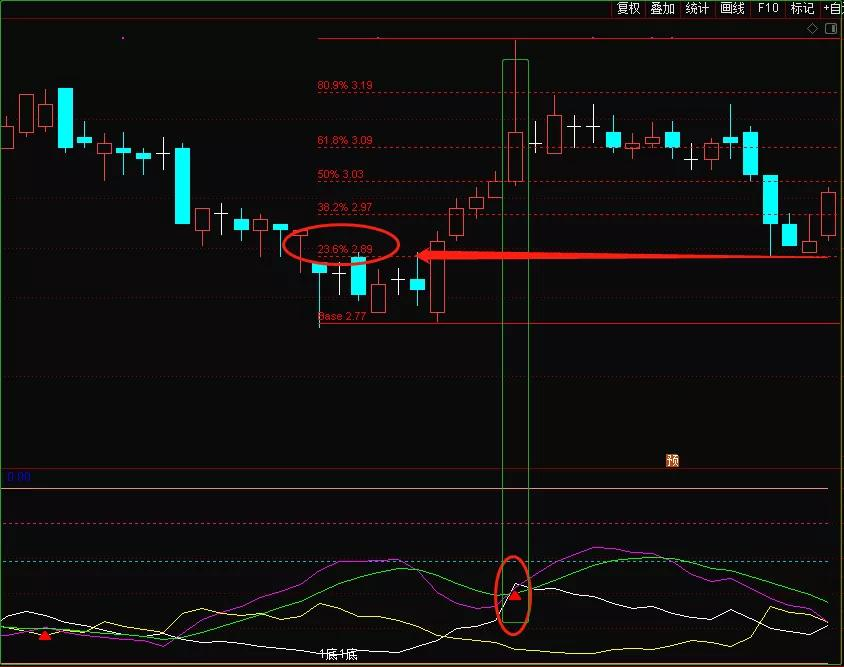[!--temp.sitename--]声明：资讯来源于互联网，属作者个人观点，仅供投资者参考，并不构成投资建议。投资者据此操作，风险自担。